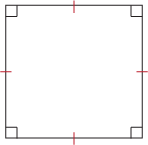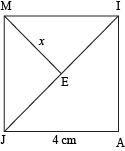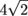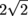Study Guide

## Squares

If you thought rectangles were special, you're in for a treat. They're called squares, and they're perfect in every way. Well, not every way because no quadrilateral is perfect. But squares are certainly the supermodels of the quadrilateral world. (Only think healthy angles instead of sickly ones.)

A square is a rectangle with four congruent sides. Or a rhombus with four congruent angles. Or a parallelogram with four congruent sides and four congruent angles. Take your pick; they're all the same. That's right: a square is a parallelogram, a rectangle, and a rhombus all rolled into one. It's all that and a bag of chips.Equilateral and equiangular mean the same thing when you're talking about triangles, but quadrilaterals are a bit pickier. A quadrilateral can be equiangular but not equilateral (a rectangle) or equilateral but not equiangular (a rhombus). A square, however, is both. All sides of a square are the same length (equilateral) and all its angles have the same measure (equiangular). This makes the square the only equilateral and equiangular quadrilateral. Of course, we can just call it a "regular quadrilateral."

### Sample Problem

A square's interior angle has a measure that's 18 times the length of its side. What is the perimeter of the square?

We know that each interior angle in a square has a measure of 90°. (Squares are equiangular and have 4 angles totaling 360°.) To find the perimeter, we need to multiply the side length of the square s by 4 because a square has four congruent sides. So basically, P = 4s.

If one angle's measure is 18 times that of the side, we know that 18s = 90. We can find the value of s by finding 90 ÷ 18. Once we've calculated s = 5, we can find P = 4s = 4(5) = 20.

### Sample Problem

If a quadrilateral is a rhombus, is it also a square?

A quadrilateral is any four-sided figure. A rhombus is a quadrilateral with four congruent sides. A square is a quadrilateral with four congruent sides and four congruent angles. So the question here becomes: must a quadrilateral with four congruent sides have four congruent angles?

The straight answer here is no. Rhombi are parallelograms, so opposite angles are congruent and consecutive ones are supplementary. If one is 90°, then they're all 90°, but where is it written that they have to be 90°? It isn't. Not anywhere we can see, anyway. So while a rhombus can be a square, it doesn't have to be.

Since squares are just rectangular rhombi (or rhomboidal rectangles), their diagonals have the same properties as those of rhombi and rectangles. Namely, a square's diagonals are congruent perpendicular bisectors of each other. Plus, they can tap dance, play the cello, and do parkour. Multi-talented little rascals, aren't they?

### Sample Problem

Quadrilateral JAIM is a square. Find the length of x.We have two ways to go about this, and both of 'em involve right triangles. We would apologize for that, but it's about time you got used to them.

First, we could look at ΔJAI and solve for the length of its hypotenuse JI. Since both the legs (JA and AI) are congruent, we know that ΔJAI is a 45-45-90 triangle with legs of 4 cm. Whether we've memorized properties of these triangles or we pull out the Pythagorean Theorem, we should get a length ofcm for JI. Since the diagonals of a square are congruent and bisect each other, we know that x is half that length, orcm ≈ 2.83 cm.

The second way is to realize that a square is also a rhombus, which means that its diagonals are perpendicular bisectors. In other words, not only does segment ME have a length of x, but EI has a length of x too. And since ∠MEI is a right angle, we have another 45-45-90 triangle: ΔMEI. We know the hypotenuse (MI = 4 cm), and we can figure out the length of a single leg, x. Whether by Pythagorean Theorem or special triangle properties, we should getcm ≈ 2.83 cm as well.

If a square falls in a forest, but nobody is there to hear it, what is the meaning of life?

We aren't going to address such terrifying existential crises like this one, but we do want to know how to tell a square is a square—regardless of the meaning of life. So, if it looks like a square and it rolls like a square, is it a square? (Have you ever tried rolling a square? Even downhill, it ain't easy.) We can tell a couple different ways.

1. If a quadrilateral is equilateral and equiangular, it's a square.
2. If a rhombus is equiangular, it's a square.
3. If a rectangle is equilateral, it's a square.

Basically, if you can prove that a quadrilateral is both a rhombus and a rectangle, you've proved that it's a square. Of course, we can use diagonals to prove the rhombus and rectangle business, but we'll leave that to you.